Example Application Form Required to Answer Question 6

Your answers should be placed into the boxes beneath each section. Some sections require an A, B, C or D answer and these can be selected using the drop down option when the box is clicked.

• Hidden

• Question 1: Type these numbers into the boxes below.

••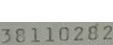••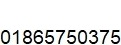••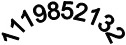••• Question 2: Which of these sets of numbers is the odd one out?

•• Question 3: Put these dates into ascending date order.• Earliest
•
•
• Latest

• Question 4: Find the smallest and largest values in the table below and type the values into the boxes.• Question 5: Please complete the following calculations.

•••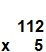•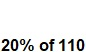• Question 6: Using the PDF you opened at the start of this test, copy the details into the following boxes.

• Question 7: Using the PDF you opened at the start of this test, if you carried out the calibration on 15 Jan 2020, which MONTH would you enter onto the calibration label?